# RS Aggarwal Solutions for Class 8 Maths Chapter 8 - Linear Equations

RS Aggarwal Solutions For Class 8 Maths Chapter 8 Linear Equations are provided here. You can download the pdf of RS Aggarwal Solutions for Class 8 Maths Chapter 8 Linear equations from the given links. RS Aggarwal Solutions helps students to get a good score in the examinations, while also providing extensive knowledge about the subject, as Class 8 is a critical stage in their academic career. Therefore, we at BYJU’S provide answers to all questions uniquely and briefly.

RS Aggarwal Solutions For Class 8 Maths also provides the foundation to higher studies. RS Aggarwal Solutions for Class 8 Maths Chapter 8 Linear Equations provides the concepts in an effective way.

## Download pdf of RS Aggarwal Solutions For Class 8 Maths Chapter 8 Linear Equations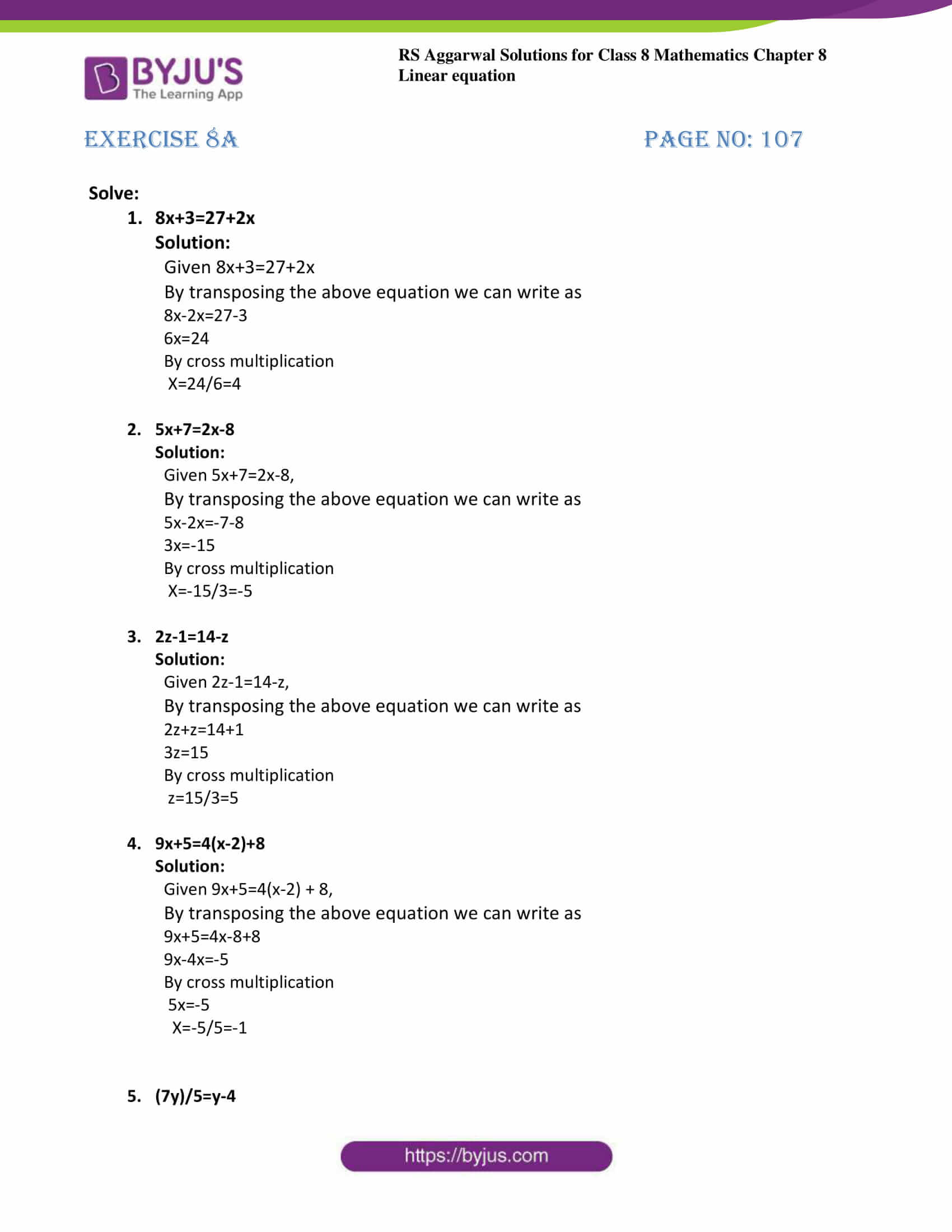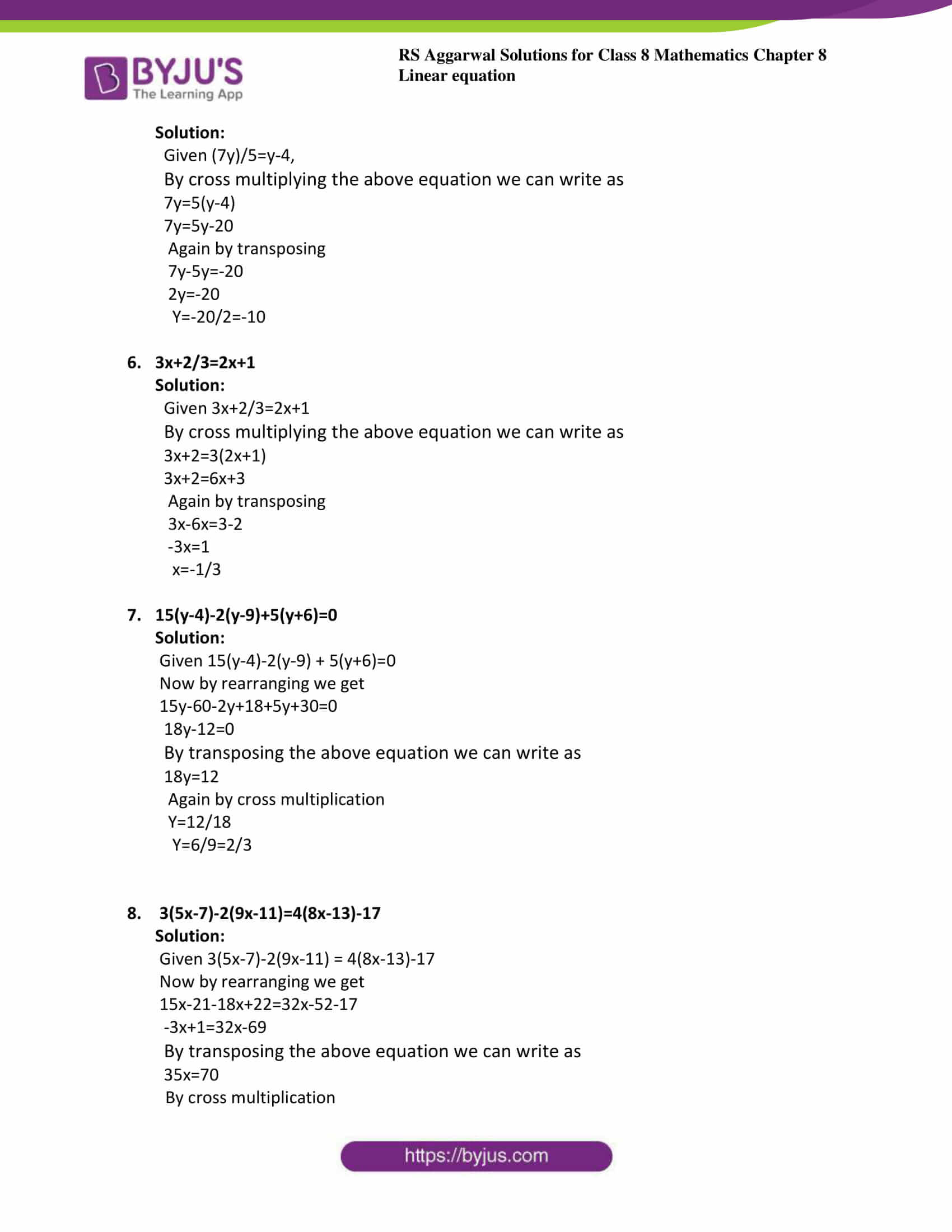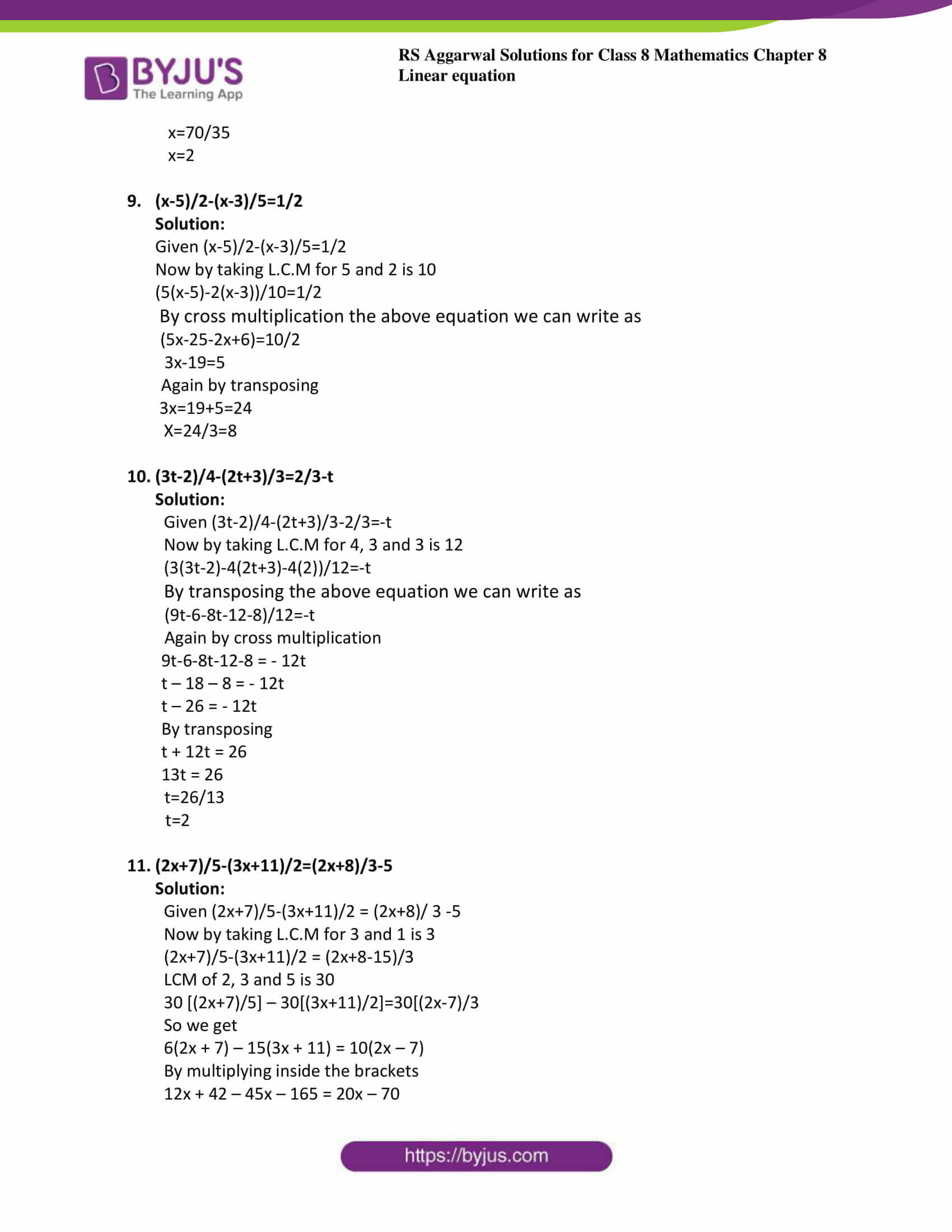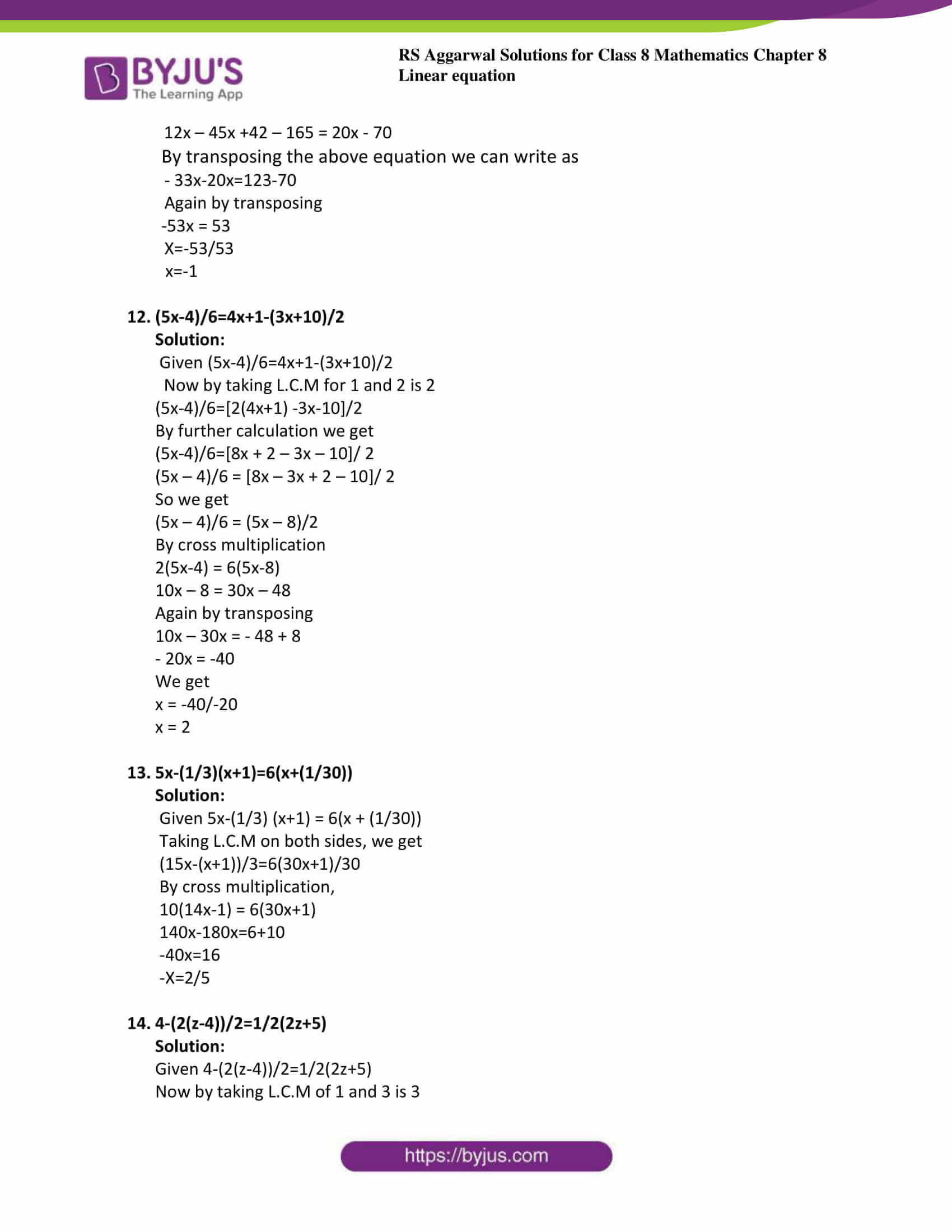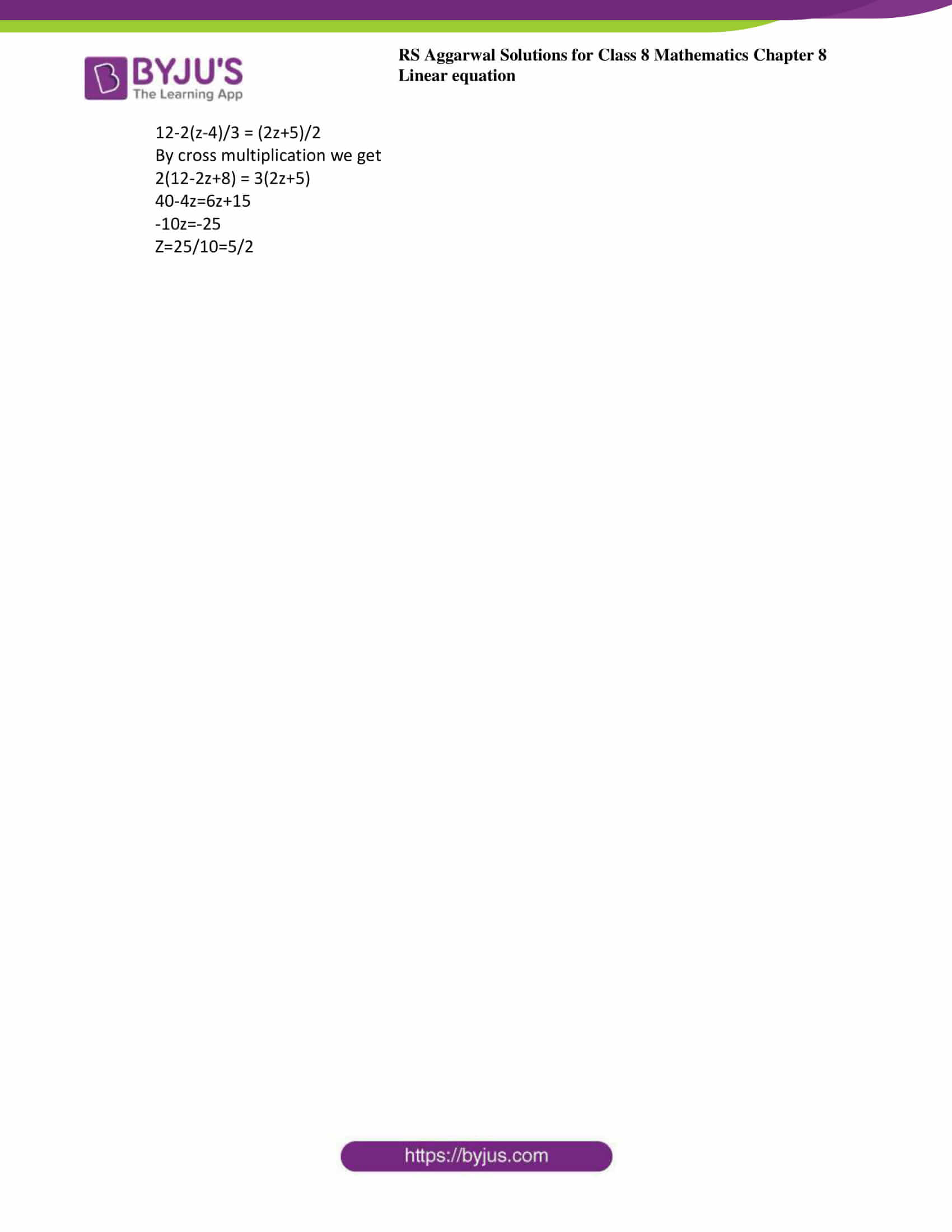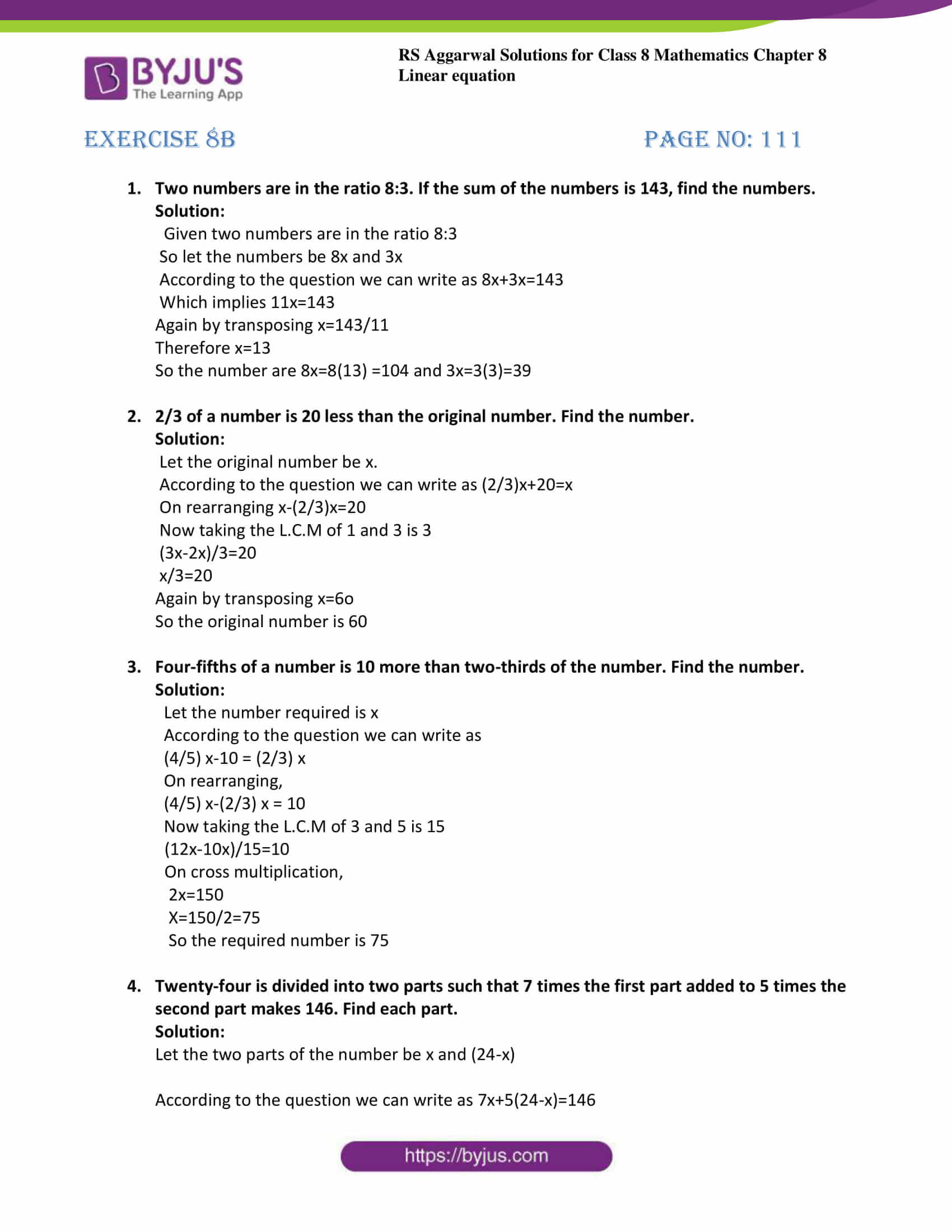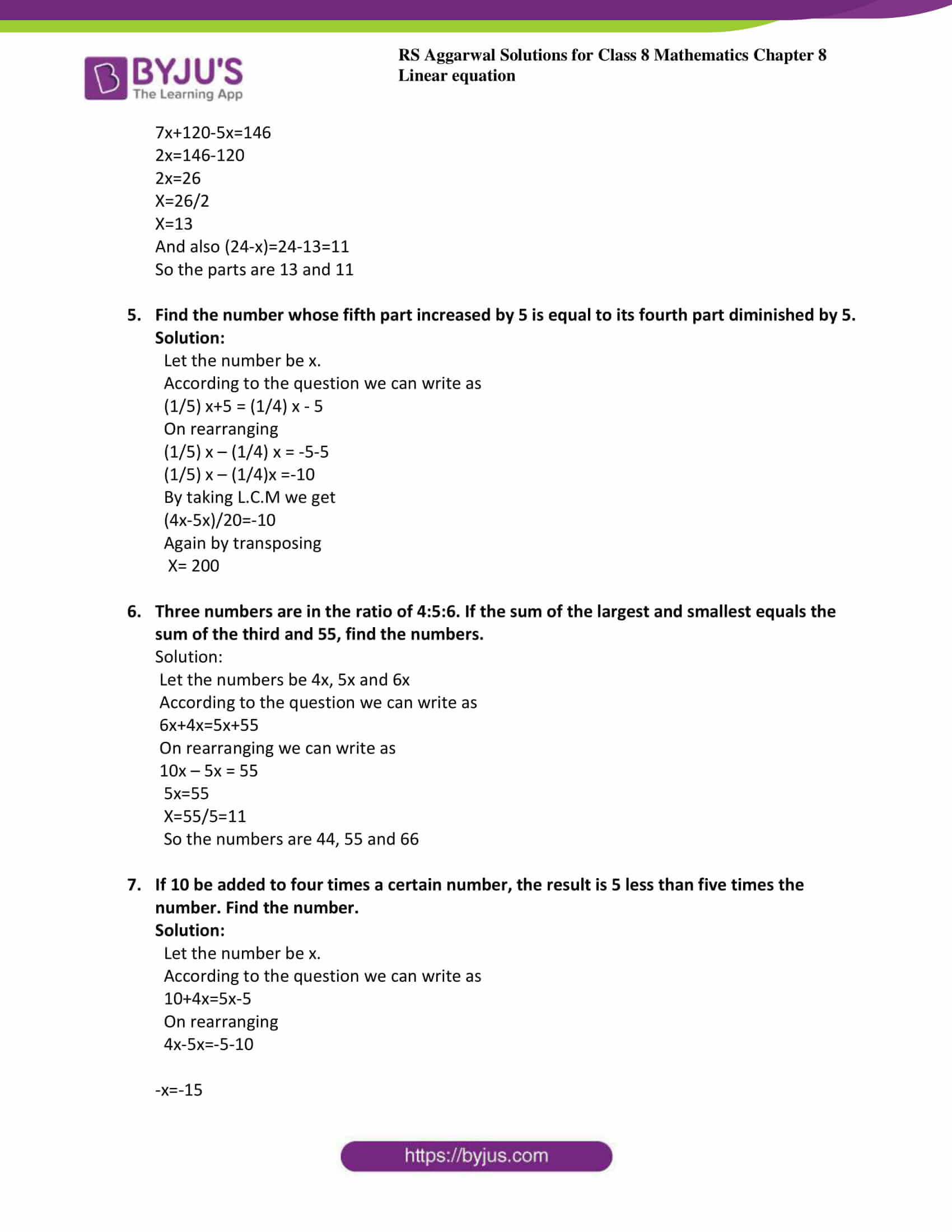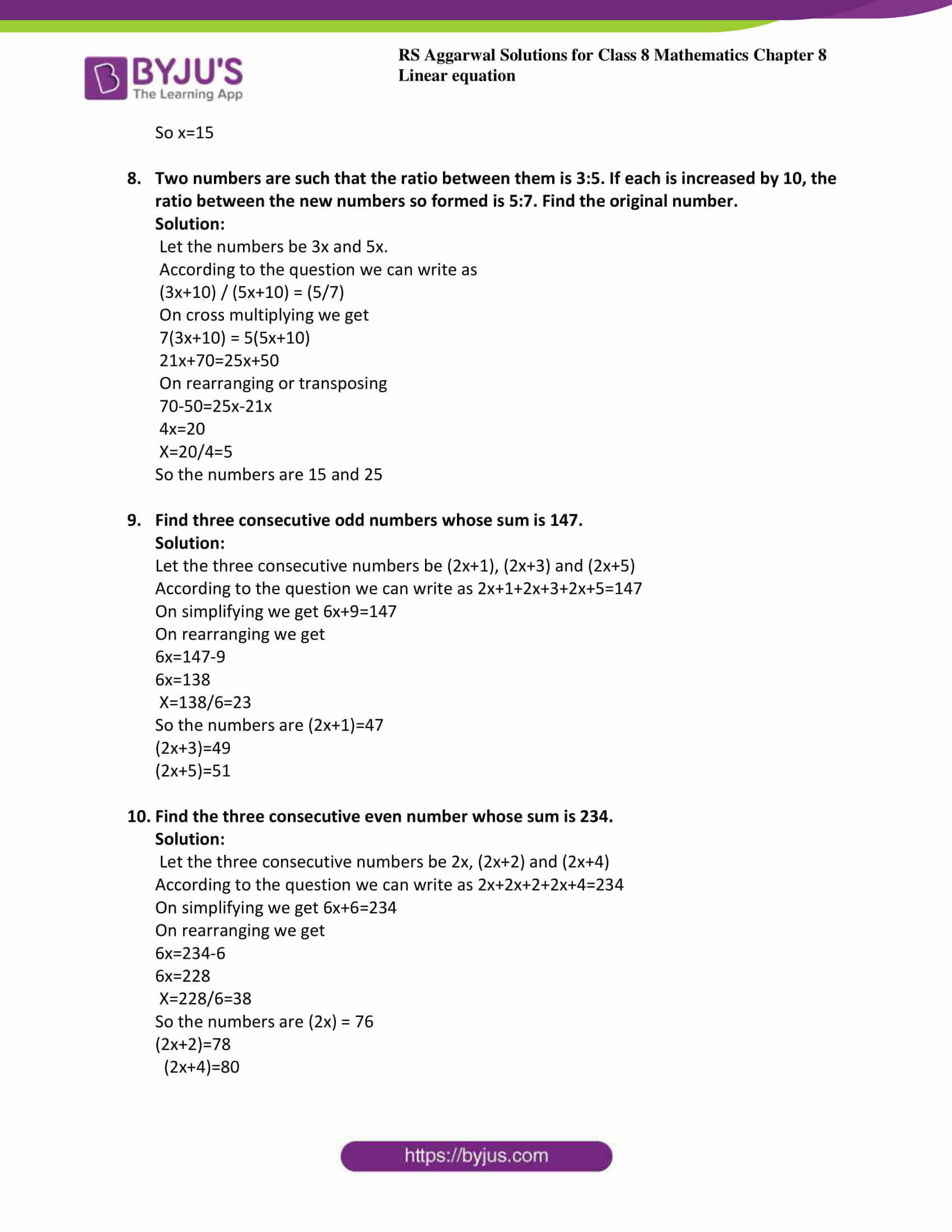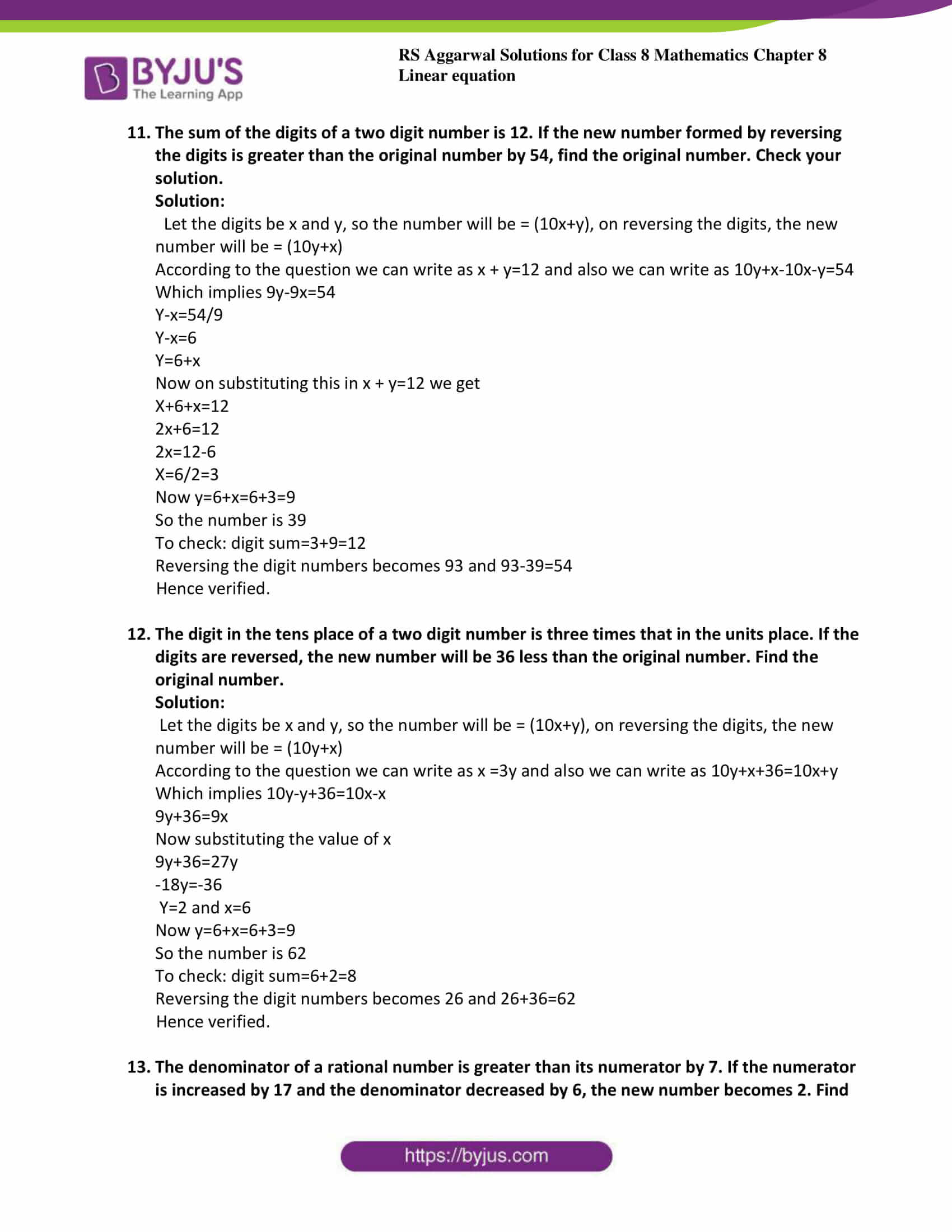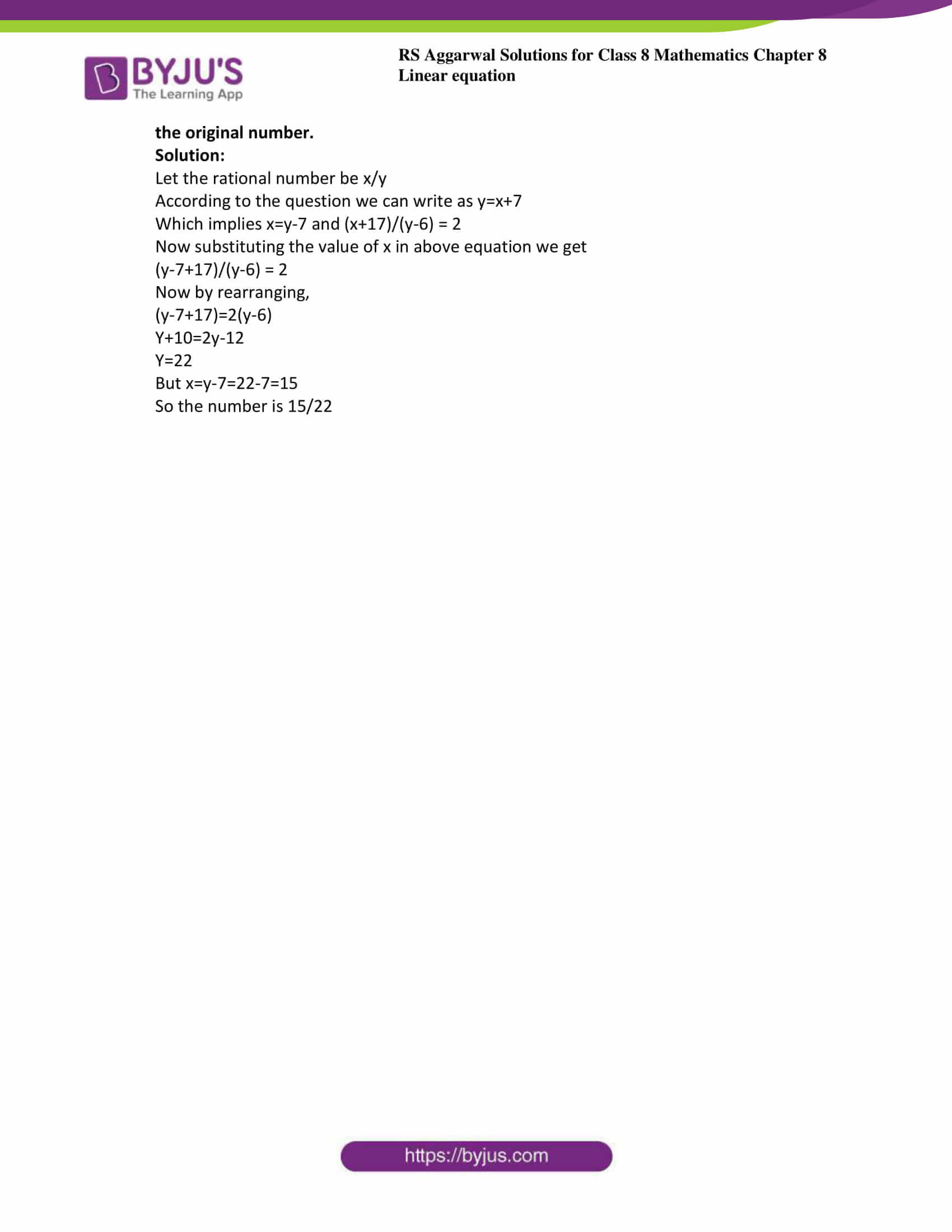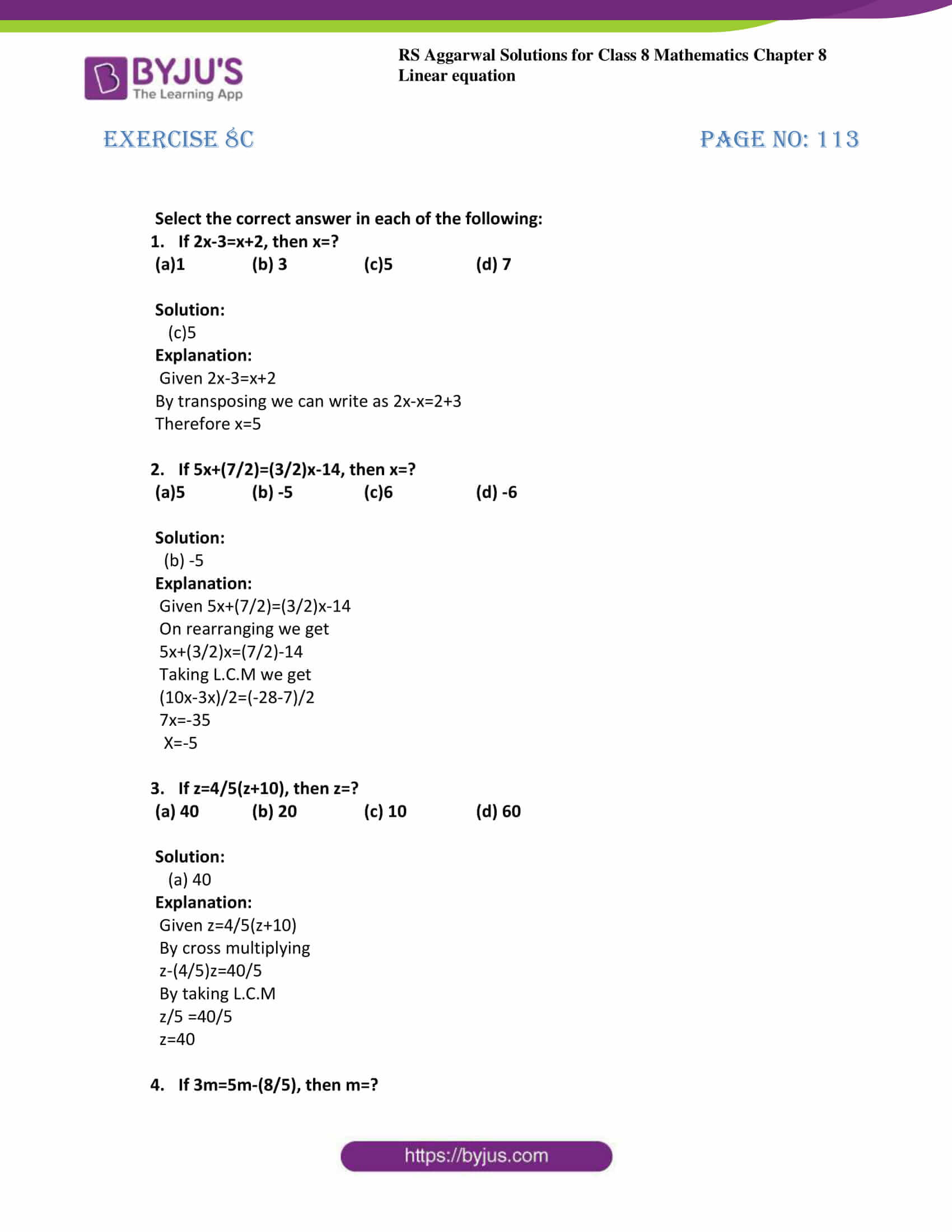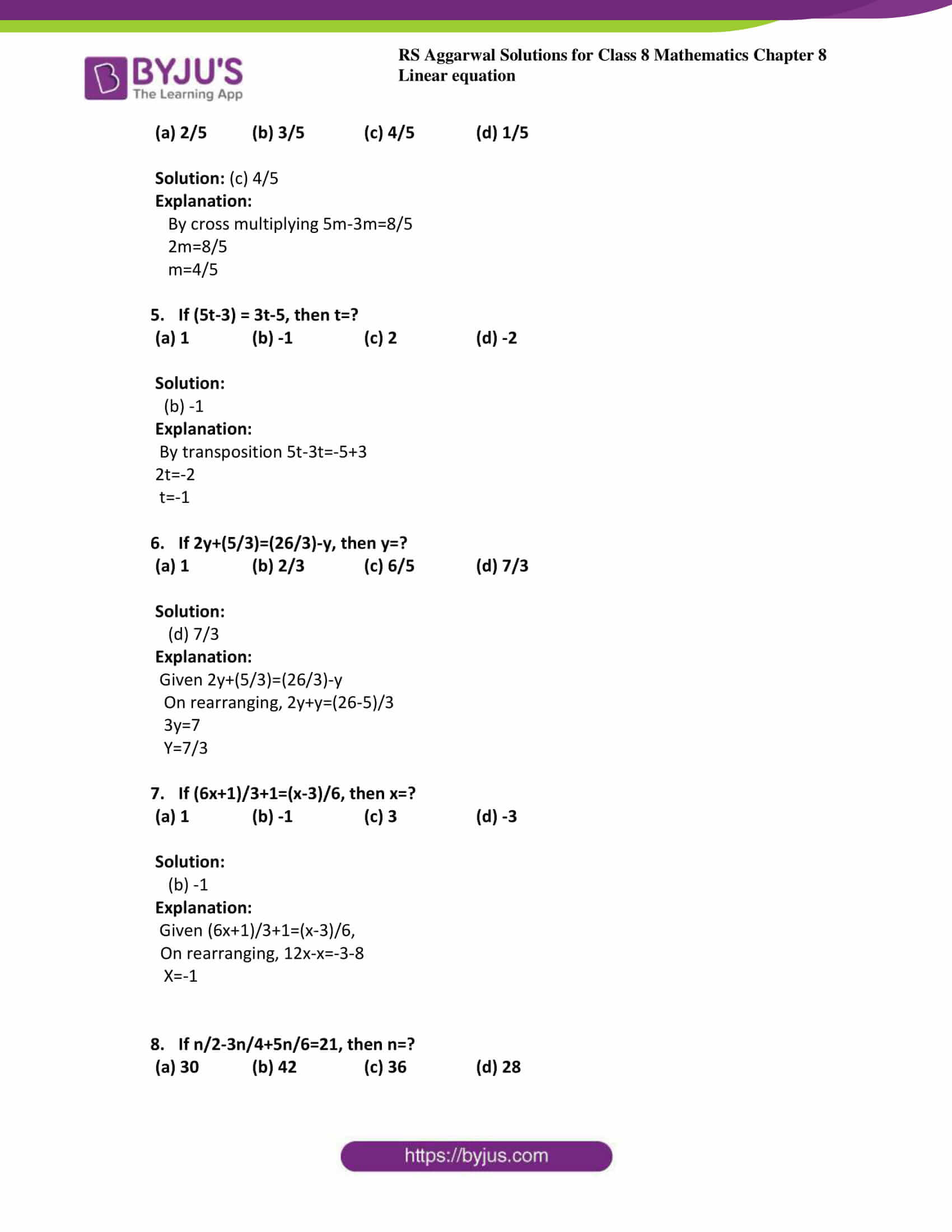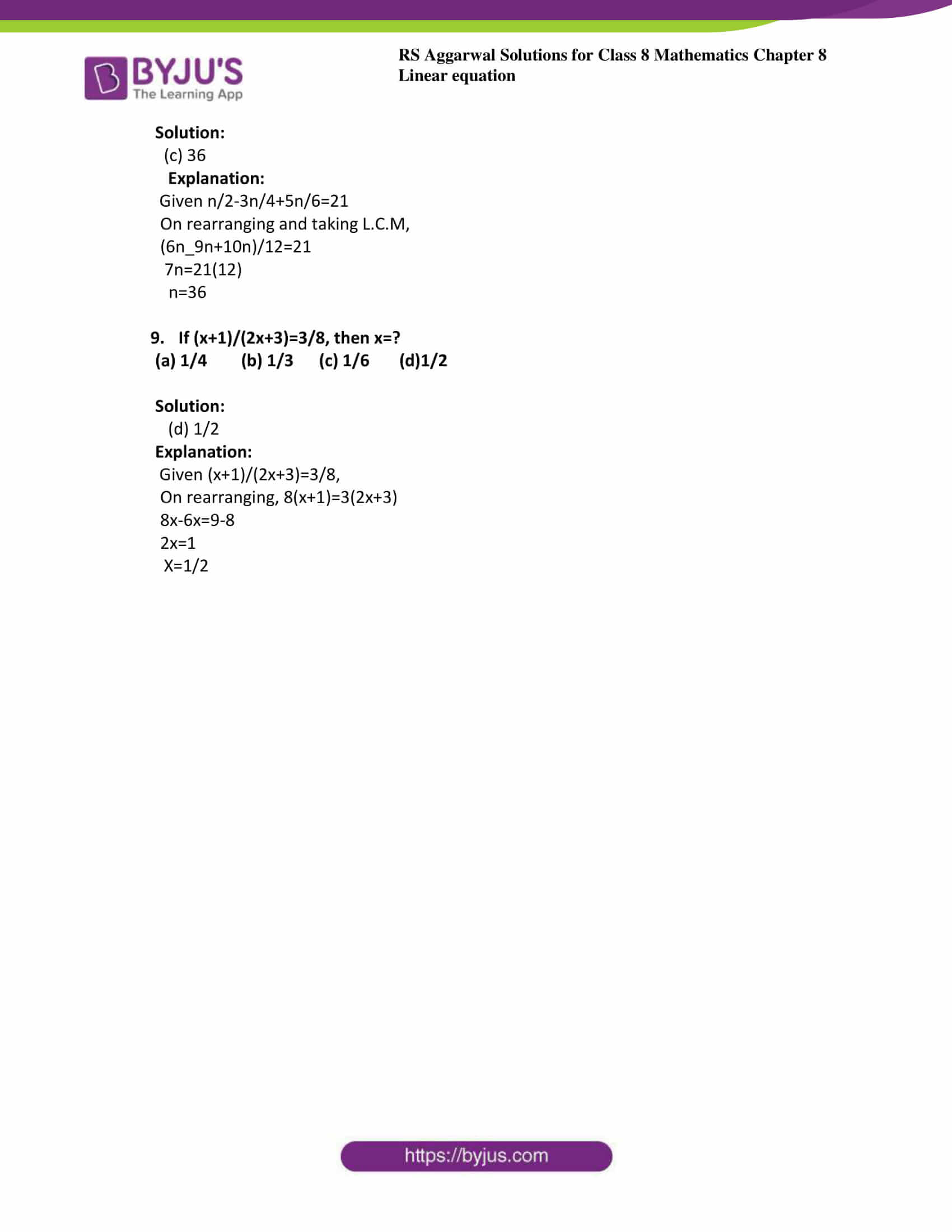## Exercise 8A

Solve:

1. 8x+3=27+2x

Solution:

Given 8x+3=27+2x

By transposing the above equation we can write as

8x-2x=27-3

6x=24

By cross multiplication

x=24/6=4

2. 5x+7=2x-8

Solution:

Given 5x+7=2x-8,

By transposing the above equation we can write as

5x-2x=-7-8

3x=-15

By cross multiplication

x=-15/3=-5

3. 2z-1=14-z

Solution:

Given 2z-1=14-z,

By transposing the above equation we can write as

2z+z=14+1

3z=15

By cross multiplication

z=15/3=5

4. 9x+5=4(x-2)+8

Solution:

Given 9x+5=4(x-2) + 8,

By transposing the above equation we can write as

9x+5=4x-8+8

9x-4x=-5

By cross multiplication

5x=-5

x=-5/5=-1

5. (7y)/5=y-4

Solution:

Given (7y)/5=y-4,

By cross multiplying the above equation we can write as

7y=5(y-4)

7y=5y-20

Again by transposing

7y-5y=-20

2y=-20

y=-20/2=-10

6. 3x+2/3=2x+1

Solution:

Given 3x+2/3=2x+1

By cross multiplying the above equation we can write as

3x+2=3(2x+1)

3x+2=6x+3

Again by transposing

3x-6x=3-2

-3x=1

x=-1/3

7. 15(y-4)-2(y-9)+5(y+6)=0

Solution:

Given 15(y-4)-2(y-9) + 5(y+6)=0

Now by rearranging we get

15y-60-2y+18+5y+30=0

18y-12=0

By transposing the above equation we can write as

18y=12

Again by cross multiplication

y=12/18

y=6/9=2/3

8. 3(5x-7)-2(9x-11)=4(8x-13)-17

Solution:

Given 3(5x-7)-2(9x-11) = 4(8x-13)-17

Now by rearranging we get

15x-21-18x+22=32x-52-17

-3x+1=32x-69

By transposing the above equation we can write as

35x=70

Again by cross multiplication

x=70/35

x=2

9. (x-5)/2-(x-3)/5=1/2

Solution:

Given (x-5)/2-(x-3)/5=1/2

Now by taking L.C.M for 5 and 2 is 10

(5(x-5)-2(x-3))/10=1/2

By cross multiplying the above equation we can write as

(5x-25-2x+6)=10/2

3x-19=5

Again by transposing

3x=19+5=24

x=24/3=8

10. (3t-2)/4-(2t+3)/3=2/3-t

Solution:

Given (3t-2)/4-(2t+3)/3-2/3=t

Now by taking L.C.M for 4, 3 and 3 is 12

(3(3t-2)-4(2t+3)-4(2))/12=-t

By transposing the above equation we can write as

(9t-6-8t-12-8)/12=-t

Again by cross multiplication

9t – 6 – 8t – 12 – 8 = – 12t

t – 18 – 8 = -12t

t – 26 = – 12t

Again by transposing

t + 12t = 26

13t = 26

t=26/13

t=2

11. (2x+7)/5-(3x+11)/2=(2x+8)/3-5

Solution:

Given (2x+7)/5-(3x+11)/2 = (2x+8)/3-5

Now by taking L.C.M for 3 and 1 is 3

(2x+7)/5-(3x+11)/2 =(2x+8-15)/3

LCM of 5, 2 and 3 is 30

30[(2x+7)/5] – 30[(3x+11)/2] = 30[(2x-7)/3]

6(2x+7) – 15(3x+11) = 10(2x-7)

By further calculation

12x+42-45x-165=20x-70

12x-45x+42-165=20x-70

-33x-123=20x-70

By transposing the above equation we can write as

-33x-20x=123-70

Again by transposing

x=-53/53

x=-1

12. (5x-4)/6=4x+1-(3x+10)/2

Solution:

Given (5x-4)/6=4x+1-(3x+10)/2

Now by taking L.C.M for 1 and 2 is 2

5x-4/6 = [2(4x+1) -3x-10]/2

By further calculation we get

5x-4/6=[8x+2-3x-10]/2

(5x-4)/6=(5x-8)/2

By cross multiplication

2(5x-4)=6(5x-8)

10x-8=30x-48

So we get

10x-30x=-48+8

-20x=-40

Again by transposing,

x=-40/-20=2

13. 5x-(1/3)(x+1)=6(x+(1/30))

Solution:

Given 5x-(1/3) (x+1) = 6(x + (1/30))

Taking L.C.M on both sides, we get

(15x-(x+1))/3=6(30x+1)/30

By cross multiplication,

10(14x-1) = 6(30x+1)

140x-180x=6+10

-40x=16

-x=2/5

14. 4-(2(z-4))/2=1/2(2z+5)

Solution:

Given 4-(2(z-4))/2=1/2(2z+5)

Now by taking L.C.M of 1 and 3 is 3

12-2(z-4)/3 = (2z+5)/2

By cross multiplication we get

2(12-2z+8) = 3(2z+5)

40-4z=6z+15

-10z=-25

z=25/10=5/2

## Exercise 8B

1. Two numbers are in the ratio 8:3. If the sum of the numbers is 143, find the numbers.

Solution:

Given two numbers are in the ratio 8:3

So let the numbers be 8x and 3x

According to the question we can write as 8x+3x=143

Which implies 11x=143

Again by transposing x=143/11

Therefore x=13

So the number are 8x=8(13) =104 and 3x=3(3)=39

2. 2/3 of a number is 20 less than the original number. Find the number.

Solution:

Let the original number be x.

According to the question we can write as (2/3)x+20=x

On rearranging x-(2/3)x=20

Now taking the L.C.M of 1 and 3 is 3

(3x-2x)/3=20

x/3=20

Again by transposing x=6o

So the original number is 60

3. Four-fifths of a number is 10 more than two-thirds of the number. Find the number.

Solution:

Let the number required is x

According to the question we can write as

(4/5) x-10 = (2/3) x

On rearranging,

(4/5) x-(2/3) x = 10

Now taking the L.C.M of 3 and 5 is 15

(12x-10x)/15=10

On cross multiplication,

2x=150

x=150/2=75

So the required number is 75

4. Twenty-four is divided into two parts such that 7 times the first part added to 5 times the second part makes 146. Find each part.

Solution:

Let the two parts of the number be x and (24-x)

According to the question we can write as 7x+5(24-x)=146

7x+120-5x=146

2x=146-120

2x=26

x=26/2

x=13

And also (24-x)=24-13=11

So the parts are 13 and 11

5. Find the number whose fifth part increased by 5 is equal to its fourth part diminished by 5.

Solution:

Let the number be x.

According to the question we can write as

(1/5) x+5 = (1/4) x – 5

On rearranging

(1/5) x – (1/4) x = -5-5

(1/5) x – (1/4)x =-10

By taking L.C.M we get

(4x-5x)/20=-10

Again by transposing

x= 200

6. Three numbers are in the ratio of 4:5:6. If the sum of the largest and smallest equals the sum of the third and 55, find the numbers.

Solution:

Let the numbers be 4x, 5x and 6x

According to the question we can write as

6x+4x=5x+55

On rearranging we can write as

10x – 5x = 55

5x=55

x=55/5=11

So the numbers are 44, 55 and 66

7. If 10 be added to four times a certain number, the result is 5 less than five times the number. Find the number.

Solution:

Let the number be x.

According to the question we can write as

10+4x=5x-5

On rearranging

4x-5x=-5-10

-x=-15

So x=15

8. Two numbers are such that the ratio between them is 3:5. If each is increased by 10, the ratio between the new numbers so formed is 5:7. Find the original number.

Solution:

Let the numbers be 3x and 5x.

According to the question we can write as

(3x+10) / (5x+10) = (5/7)

On cross multiplying we get

7(3x+10) = 5(5x+10)

21x+70=25x+50

On rearranging or transposing

70-50=25x-21x

4x=20

x=20/4=5

So the numbers are 15 and 25

9. Find three consecutive odd numbers whose sum is 147.

Solution:

Let the three consecutive numbers be (2x+1), (2x+3) and (2x+5)

According to the question we can write as 2x+1+2x+3+2x+5=147

On simplifying we get 6x+9=147

On rearranging we get

6x=147-9

6x=138

x=138/6=23

So the numbers are (2x+1)=47

(2x+3)=49

(2x+5)=51

10. Find the three consecutive even number whose sum is 234.

Solution:

Let the three consecutive numbers be 2x, (2x+2) and (2x+4)

According to the question we can write as 2x+2x+2+2x+4=234

On simplifying we get 6x+6=234

On rearranging we get

6x=234-6

6x=228

x=228/6=38

So the numbers are (2x) = 76

(2x+2)=78

(2x+4)=80

11. The sum of the digits of a two digit number is 12. If the new number formed by reversing the digits is greater than the original number by 54, find the original number. Check your solution.

Solution:

Let the digits be x and y, so the number will be = (10x+y), on reversing the digits, the new number will be = (10y+x)

According to the question we can write as x + y=12 and also we can write as 10y+x-10x-y=54

Which implies 9y-9x=54

y-x=54/9

y-x=6

y=6+x

Now on substituting this in x + y=12 we get

x+6+x=12

2x+6=12

2x=12-6

x=6/2=3

Now y=6+x=6+3=9

So the number is 39

To check: digit sum=3+9=12

Reversing the digit numbers becomes 93 and 93-39=54

Hence verified.

12. The digit in the tens place of a two digit number is three times that in the units place. If the digits are reversed, the new number will be 36 less than the original number. Find the original number.

Solution:

Let the digits be x and y, so the number will be = (10x+y), on reversing the digits, the new number will be = (10y+x)

According to the question we can write as x =3y and also we can write as 10y+x+36=10x+y

Which implies 10y-y+36=10x-x

9y+36=9x

Now substituting the value of x

9y+36=27y

-18y=-36

y=2 and x=6

Now y=6+x=6+3=9

So the number is 62

To check: digit sum=6+2=8

Reversing the digit numbers becomes 26 and 26+36=62

Hence verified.

13. The denominator of a rational number is greater than its numerator by 7. If the numerator is increased by 17 and the denominator decreased by 6, the new number becomes 2. Find the original number.

Solution:

Let the rational number be x/y

According to the question we can write as y=x+7

Which implies x=y-7 and (x+17)/(y-6) = 2

Now substituting the value of x in above equation we get

(y-7+17)/(y-6) = 2

Now by rearranging,

(y-7+17)=2(y-6)

y+10=2y-12

y=22

But x=y-7=22-7=15

So the number is 15/22

## Exercise 8C

Select the correct answer in each of the following:

1. If 2x-3=x+2, then x=?

(a)1 (b) 3 (c)5 (d) 7

Solution:

(c)5

Explanation:

Given 2x-3=x+2

By transposing we can write as 2x-x=2+3

Therefore x=5

2. If 5x+(7/2)=(3/2)x-14, then x=?

(a)5 (b) -5 (c)6 (d) -6

Solution:

(b) -5

Explanation:

Given 5x+(7/2)=(3/2)x-14

On rearranging we get

5x+(3/2)x=(7/2)-14

Taking L.C.M we get

(10x-3x)/2=(-28-7)/2

7x=-35

x=-5

3. If z=4/5(z+10), then z=?

(a) 40 (b) 20 (c) 10 (d) 60

Solution:

(a) 40

Explanation:

Given z=4/5(z+10)

By cross multiplying

z-(4/5)z=40/5

By taking L.C.M

z/5 =40/5

z=40

4. If 3m=5m-(8/5), then m=?

(a) 2/5 (b) 3/5 (c) 4/5 (d) 1/5

Solution: (c) 4/5

Explanation:

By cross multiplying 5m-3m=8/5

2m=8/5

m=4/5

5. If (5t-3) = 3t-5, then t=?

(a) 1 (b) -1 (c) 2 (d) -2

Solution:

(b) -1

Explanation:

By transposition 5t-3t=-5+3

2t=-2

t=-1

6. If 2y+(5/3)=(26/3)-y, then y=?

(a) 1 (b) 2/3 (c) 6/5 (d) 7/3

Solution:

(d) 7/3

Explanation:

Given 2y+(5/3)=(26/3)-y

On rearranging, 2y+y=(26-5)/3

3y=7

y=7/3

7. If (6x+1)/3+1=(x-3)/6, then x=?

(a) 1 (b) -1 (c) 3 (d) -3

Solution:

(b) -1

Explanation:

Given (6x+1)/3+1=(x-3)/6,

On rearranging, 12x-x=-3-8

x=-1

8. If n/2-3n/4+5n/6=21, then n=?

(a) 30 (b) 42 (c) 36 (d) 28

Solution:

(c) 36

Explanation:

Given n/2-3n/4+5n/6=21

On rearranging and taking L.C.M,

(6n_9n+10n)/12=21

7n=21(12)

n=36

9. If (x+1)/(2x+3)=3/8, then x=?

(a) 1/4 (b) 1/3 (c) 1/6 (d)1/2

Solution:

(d) 1/2

Explanation:

Given (x+1)/(2x+3)=3/8,

On rearranging, 8(x+1)=3(2x+3)

8x-6x=9-8

2x=1

x=1/2

## RS Aggarwal Solutions for Class 8 Maths Chapter 8- Linear Equations

Chapter 8, Linear Equations, contains 3 exercises. RS Aggarwal Solutions given here contains the answers to all the questions present in these exercises. Let us have a look at some of the concepts that are being discussed in this chapter.

• Definition of Equation

• Rules for solving linear Equations

• Definition of Transposing

• Applications of Linear Equations

Exercise 8A

Exercise 8B

Exercise 8C

### Chapter Brief of RS Aggarwal Solutions for Class 8 Maths Chapter 8 – Linear Equations

The RS Aggarwal Solutions for Class 8 Maths Chapter 8 – Linear Equation deals with the definition of an equation, rules for solving linear equation, how to do transposing and also about cross multiplication. By using these rules we can find the unknown present in the given equation. It is helpful to solve verbally given problems in terms of equations. It is also helpful in solving problems related to geometry.